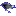# functions

This category contains 16 nodes.

##### GaussianProcessesDeprecated

Implements Gaussian Processes for regression without hyperparameter-tuning.

##### IsotonicRegressionDeprecated

Learns an isotonic regression model.

##### LeastMedSqDeprecated

Implements a least median sqaured linear regression utilising the existing weka LinearRegression class to form predictions.

##### LinearRegressionDeprecated

Class for using linear regression for prediction.

##### LogisticDeprecated

Class for building and using a multinomial logistic regression model with a ridge estimator.

##### MultilayerPerceptronDeprecated

A Classifier that uses backpropagation to classify instances.

##### PaceRegressionDeprecated

Class for building pace regression linear models and using them for prediction.

##### PLSClassifierDeprecated

A wrapper classifier for the PLSFilter, utilizing the PLSFilter's ability to perform predictions.

##### RBFNetworkDeprecated

Class that implements a normalized Gaussian radial basisbasis function network.

##### SimpleLinearRegressionDeprecated

Learns a simple linear regression model.